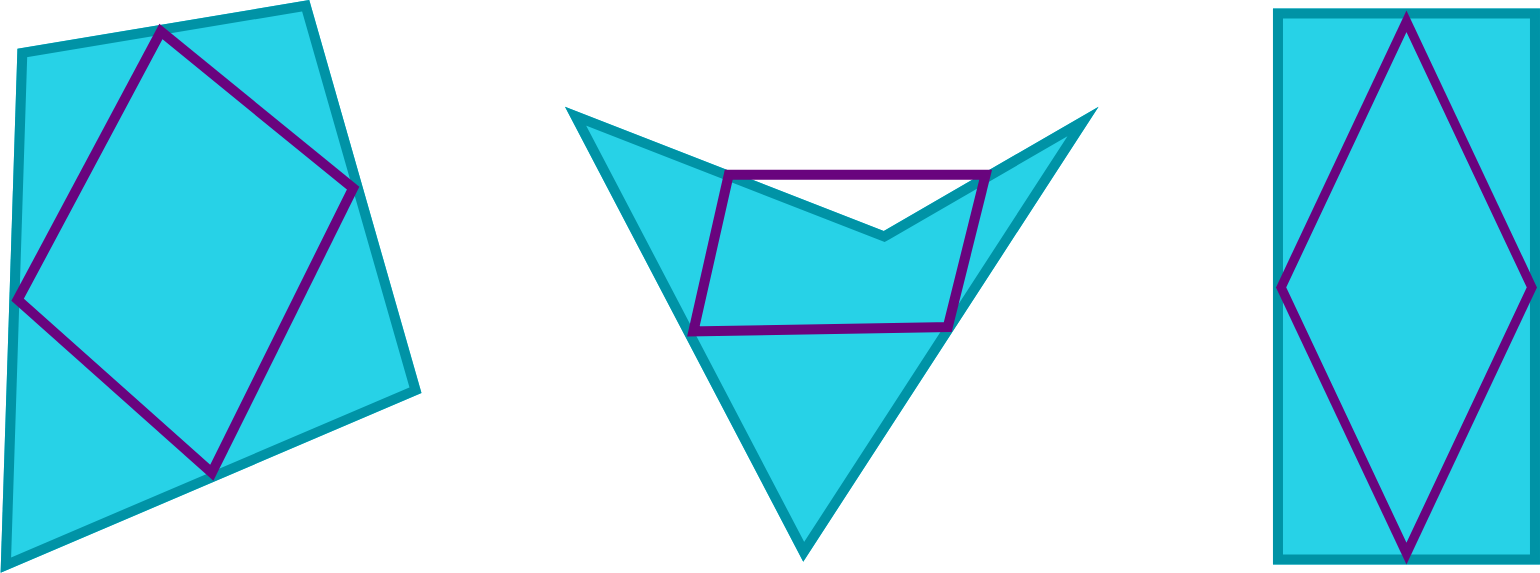Geometry

# Properties of Squares

True or False?

Connecting the consecutive midpoints of the four sides of any simple quadrilateral makes a parallelogram.Square $ABCD$ has side length equal to $12$. $E$ is the midpoint of $AD$ and $F$ is the midpoint of $DC$. What is the area of quadrilateral $BEFC$?

$ABCD$ is a square such that $[ABCD] = 120$. $E, F, G, H$ are the midpoints of $AB, BC, CD$ and $DA$ respectively. What is $[EFGH]$?

Details and assumptions

$[PQRS]$ denotes the area of figure $PQRS$.

Square $ABCD$ has $M$ as the midpoint of $AB$, $N$ as the midpoint of $BC$, $P$ as the midpoint of $CD$ and $Q$ as the midpoint of $MP$. If $[AMNPDQ] = 50$, what is the value of $[ABCD]$?

Details and assumptions

$[PQRS]$ refers to the area of figure $PQRS$.

How many sides of a square have equal length?

×